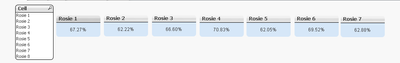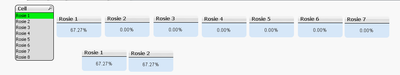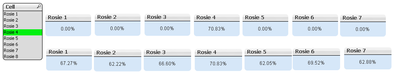# QlikView App Dev

Discussion Board for collaboration related to QlikView App Development.

Announcements
QlikWorld 2023, a live, in-person thrill ride. Save \$300 before February 6: REGISTER NOW!
cancel
Showing results for
Did you mean:Creator II

## Set Analysis With Variable

Hi All,

I have created some text boxes based on the Cell Field Values. Below is the expression.

=Num(Sum(Aggr(If(Cell = 'Rosie 1', \$(vOEE)),Cell)),'#,##0.00%')

Without Selection Cell Field Selection values are showing in all text boxes.if i select one value from Cell field, selected value is showing in text boxes where as remaining its showing as 0.My requirement is whatever value selected from Cell , all text boxes should be showing their corresponding values.

Labels (1)
• ### Application Development

1 Solution

Accepted SolutionsMVP

Hi Dinesh,

Try like belowNum(\$(vAvailability(\$1)) * \$(vPerformance(\$1)) * \$(vQuality(\$1)),'#,##0.00%')

Thanks & Regards,
Mayil Vahanan R
8 RepliesMVP

maybe something like

``Num(Sum({\$<Cell={'Rosie 1'}>} \$(vOEE)),'#,##0.00%')``

what is the content of variable vOEE?

hope this helps

regards

MarcoCreator II
Author

Hi Macro,

vOEE is

Num(If(Sum([Net Available]) <> 0, Sum(Running)/Sum([Net Available]), 0),'#,##0.00%') *

Num(If(Sum([Pieces per Minute]) * Sum(Running) <> 0, Sum([Actual Production (Pieces)])/(Sum([Pieces per Minute]*Running)),0),'#,##0.00%') *

Num(If(Sum([Actual Production (Pieces)] ) <> 0, (Sum([Actual Production (Pieces)]) - Sum(Reject))/Sum([Actual Production (Pieces)]),0),'#,##0.00%')

Note: I want to extract the particular value from the variable. But we can use set expression in the above formula.

Regards,

DineshMVP

HI Dinesh,

Change the vOEE  like below

Num(If(Sum({<\$1>}[Net Available]) <> 0, Sum({<\$1>}Running)/Sum({<\$1>}[Net Available]), 0),'#,##0.00%') *

Num(If(Sum({<\$1>}[Pieces per Minute]) * Sum({<\$1>}Running) <> 0, Sum({<\$1>}[Actual Production (Pieces)])/(Sum({<\$1>}[Pieces per Minute]*Running)),0),'#,##0.00%') *

Num(If(Sum({<\$1>}[Actual Production (Pieces)] ) <> 0, (Sum({<\$1>}[Actual Production (Pieces)]) - Sum({<\$1>}Reject))/Sum({<\$1>}[Actual Production (Pieces)]),0),'#,##0.00%')

Try like below

=\$(vOEE(Cell={'Rosie 1'}))

Thanks & Regards,
Mayil Vahanan RCreator II
Author

Hi MayilVahanan,

I have tried your logic but its showing without any selection overall OEE.If i Select the value from Cell as Rosie 1 both values are same it means for Rosie 2 showing same value.

Rosie 1 Text Box: =\$(vOEE(Cell = {'Rosie 1'}))

Rosie 2 Text Box: =\$(vOEE(Cell = {'Rosie 2'})) but the real value is 62.22% as given the above image.

Thanks & Regard,

Dinesh.MVP

Hi Dinesh

Can you send  the sample file..

Thanks & Regards,
Mayil Vahanan RCreator II
Author

Hi,

PFA...MVP

Hi Dinesh,

Try like belowNum(\$(vAvailability(\$1)) * \$(vPerformance(\$1)) * \$(vQuality(\$1)),'#,##0.00%')

Thanks & Regards,
Mayil Vahanan RCreator II
Author

Hi MayilVahanan,

Its working fine.Thanks & Regards,

Dinesh Kumar.Community Browser